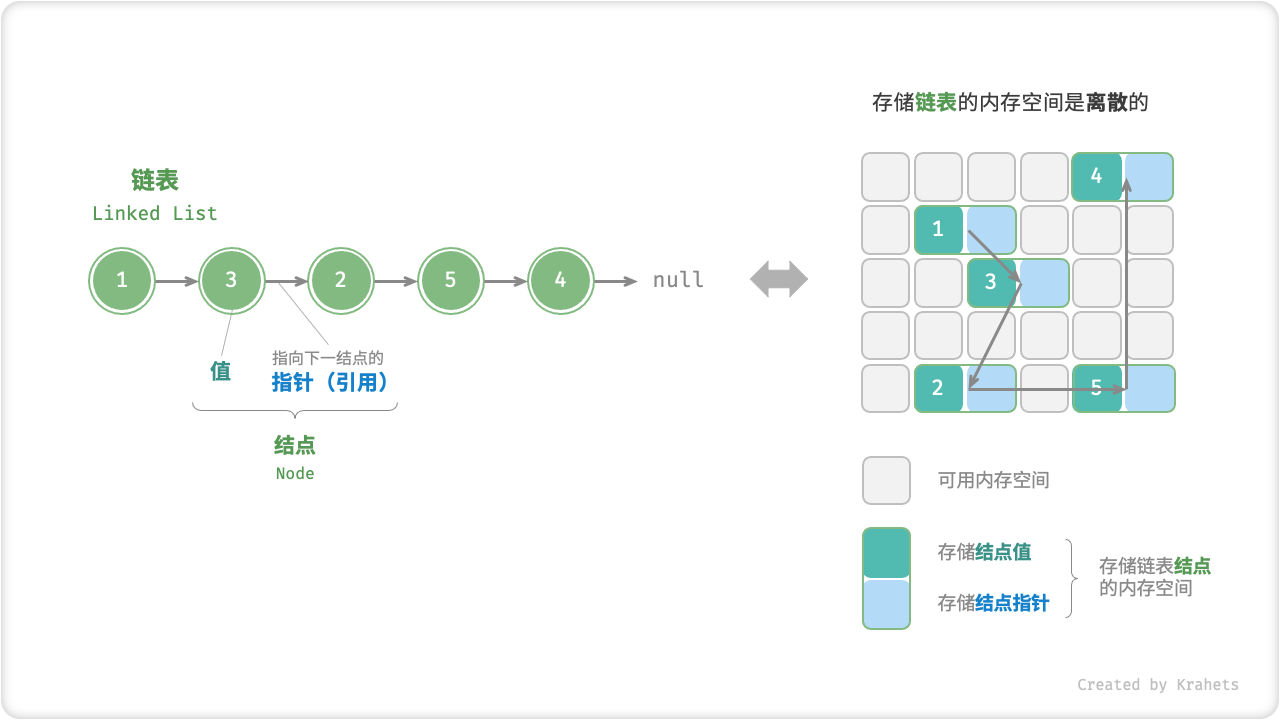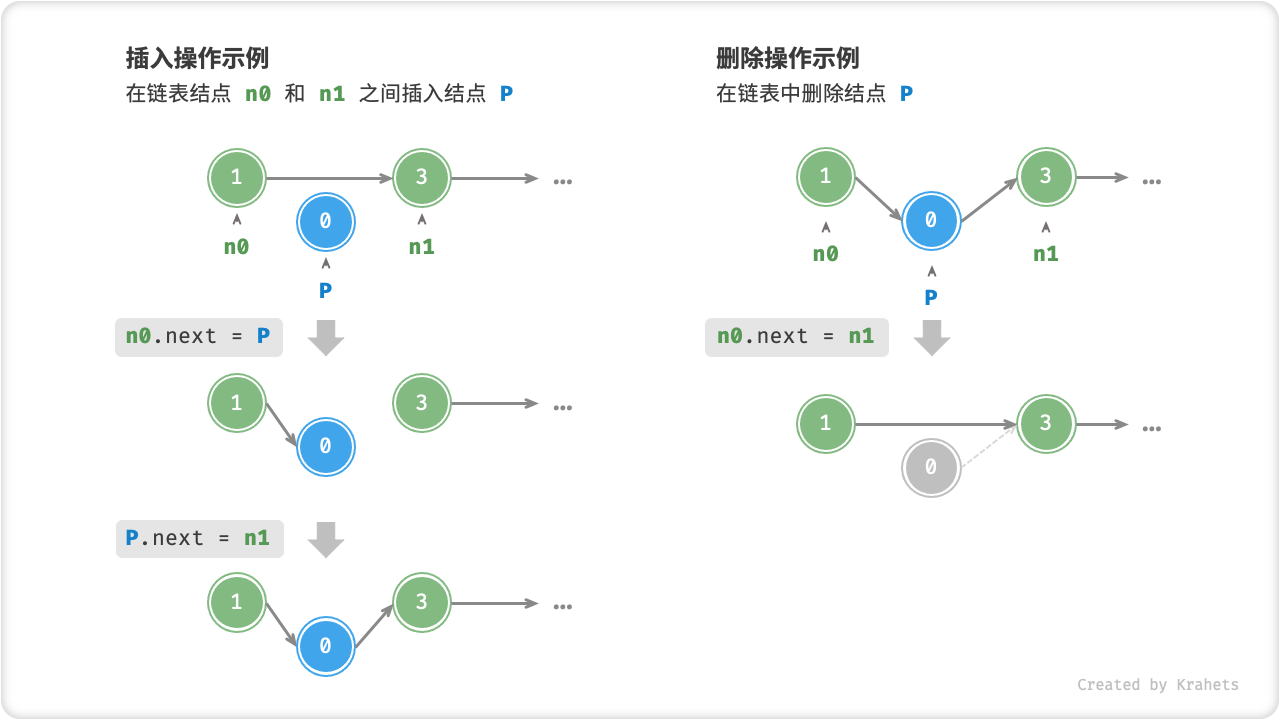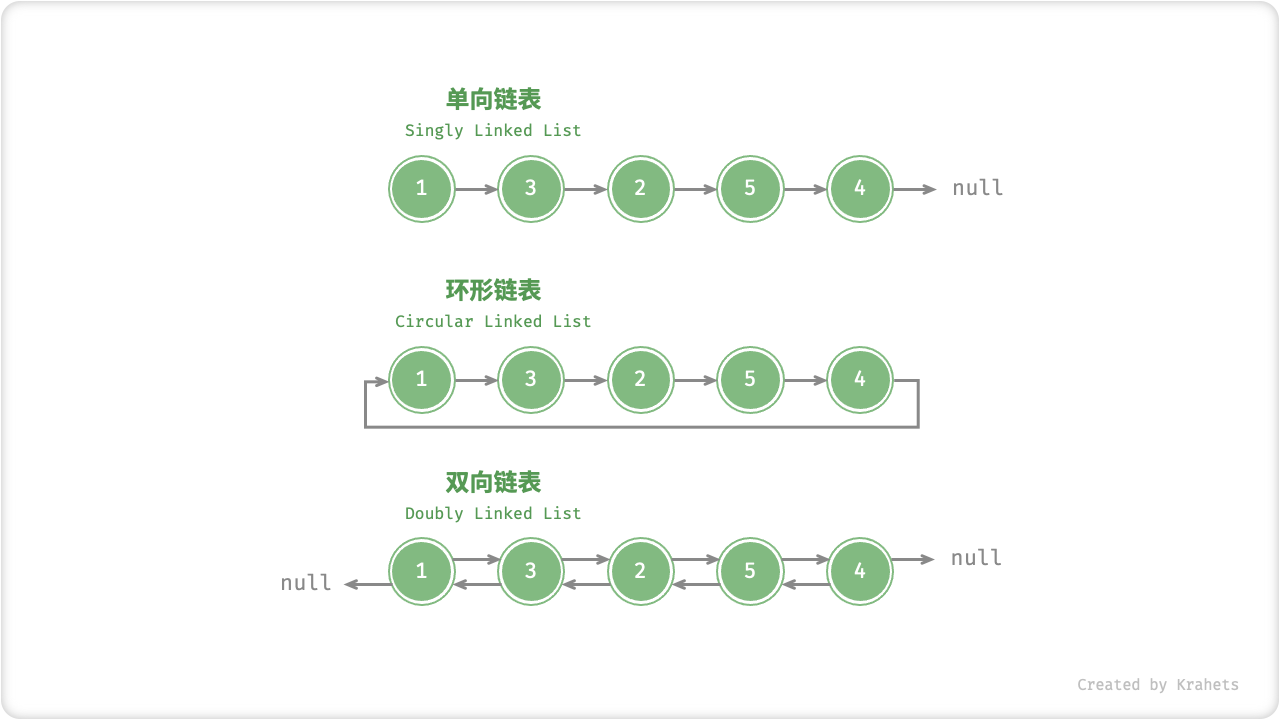# 链表¶Fig. 链表定义与存储方式

/* 链表结点类 */
class ListNode {
int val;        // 结点值
ListNode next;  // 指向下一结点的指针（引用）
ListNode(int x) { val = x; }  // 构造函数
}

/* 链表结点结构体 */
struct ListNode {
int val;         // 结点值
ListNode *next;  // 指向下一结点的指针（引用）
ListNode(int x) : val(x), next(nullptr) {}  // 构造函数
};

""" 链表结点类 """
class ListNode:
def __init__(self, x):
self.val = x      # 结点值
self.next = None  # 指向下一结点的指针（引用）



/* 链表结点结构体 */
class ListNode {
val;
next;
constructor(val, next) {
this.val = (val === undefined ? 0 : val);       // 结点值
this.next = (next === undefined ? null : next); // 指向下一结点的引用
}
}

/* 链表结点结构体 */
class ListNode {
val: number;
next: ListNode | null;
constructor(val?: number, next?: ListNode | null) {
this.val = val === undefined ? 0 : val;        // 结点值
this.next = next === undefined ? null : next;  // 指向下一结点的引用
}
}



// 链表结点类
class ListNode
{
int val;         // 结点值
ListNode next;   // 指向下一结点的引用
ListNode(int x) => val = x;  //构造函数
}


Tip

/* 初始化链表 1 -> 3 -> 2 -> 5 -> 4 */
// 初始化各个结点
ListNode n0 = new ListNode(1);
ListNode n1 = new ListNode(3);
ListNode n2 = new ListNode(2);
ListNode n3 = new ListNode(5);
ListNode n4 = new ListNode(4);
// 构建引用指向
n0.next = n1;
n1.next = n2;
n2.next = n3;
n3.next = n4;

/* 初始化链表 1 -> 3 -> 2 -> 5 -> 4 */
// 初始化各个结点
ListNode* n0 = new ListNode(1);
ListNode* n1 = new ListNode(3);
ListNode* n2 = new ListNode(2);
ListNode* n3 = new ListNode(5);
ListNode* n4 = new ListNode(4);
// 构建引用指向
n0->next = n1;
n1->next = n2;
n2->next = n3;
n3->next = n4;

""" 初始化链表 1 -> 3 -> 2 -> 5 -> 4 """
# 初始化各个结点
n0 = ListNode(1)
n1 = ListNode(3)
n2 = ListNode(2)
n3 = ListNode(5)
n4 = ListNode(4)
# 构建引用指向
n0.next = n1
n1.next = n2
n2.next = n3
n3.next = n4



/* 初始化链表 1 -> 3 -> 2 -> 5 -> 4 */
// 初始化各个结点
const n0 = new ListNode(1);
const n1 = new ListNode(3);
const n2 = new ListNode(2);
const n3 = new ListNode(5);
const n4 = new ListNode(4);
// 构建引用指向
n0.next = n1;
n1.next = n2;
n2.next = n3;
n3.next = n4;

/* 初始化链表 1 -> 3 -> 2 -> 5 -> 4 */
// 初始化各个结点
const n0 = new ListNode(1);
const n1 = new ListNode(3);
const n2 = new ListNode(2);
const n3 = new ListNode(5);
const n4 = new ListNode(4);
// 构建引用指向
n0.next = n1;
n1.next = n2;
n2.next = n3;
n3.next = n4;



// 初始化链表 1 -> 3 -> 2 -> 5 -> 4
// 初始化各结点
n0 = new ListNode(1);
n1 = new ListNode(3);
n2 = new ListNode(2);
n3 = new ListNode(5);
n4 = new ListNode(4);
// 构建引用指向
n0.next = n1;
n1.next = n2;
n2.next = n3;
n3.next = n4;


## 链表优点¶Fig. 在链表中插入与删除结点

/* 在链表的结点 n0 之后插入结点 P */
void insert(ListNode n0, ListNode P) {
ListNode n1 = n0.next;
n0.next = P;
P.next = n1;
}

/* 删除链表的结点 n0 之后的首个结点 */
void remove(ListNode n0) {
if (n0.next == null)
return;
// n0 -> P -> n1
ListNode P = n0.next;
ListNode n1 = P.next;
n0.next = n1;
}

/* 在链表的结点 n0 之后插入结点 P */
void insert(ListNode* n0, ListNode* P) {
ListNode* n1 = n0->next;
n0->next = P;
P->next = n1;
}

/* 删除链表的结点 n0 之后的首个结点 */
void remove(ListNode* n0) {
if (n0->next == nullptr)
return;
// n0 -> P -> n1
ListNode* P = n0->next;
ListNode* n1 = P->next;
n0->next = n1;
}

""" 在链表的结点 n0 之后插入结点 P """
def insert(n0, P):
n1 = n0.next
n0.next = P
P.next = n1

""" 删除链表的结点 n0 之后的首个结点 """
def remove(n0):
if not n0.next:
return
# n0 -> P -> n1
P = n0.next
n1 = P.next
n0.next = n1



/* 在链表的结点 n0 之后插入结点 P */
function insert(n0, P) {
let n1 = n0.next;
n0.next = P;
P.next = n1;
}

/* 删除链表的结点 n0 之后的首个结点 */
function remove(n0) {
if (!n0.next)
return;
// n0 -> P -> n1
let P = n0.next;
let n1 = P.next;
n0.next = n1;
}

/* 在链表的结点 n0 之后插入结点 P */
function insert(n0: ListNode, P: ListNode): void {
const n1 = n0.next;
n0.next = P;
P.next = n1;
}
/* 删除链表的结点 n0 之后的首个结点 */
function remove(n0: ListNode): void {
if (!n0.next) {
return;
}
// n0 -> P -> n1
const P = n0.next;
const n1 = P.next;
n0.next = n1;
}



// 在链表的结点 n0 之后插入结点 P
void Insert(ListNode n0, ListNode P)
{
ListNode n1 = n0.next;
n0.next = P;
P.next = n1;
}

// 删除链表的结点 n0 之后的首个结点
void Remove(ListNode n0)
{
if (n0.next == null)
return;
// n0 -> P -> n1
ListNode P = n0.next;
ListNode n1 = P.next;
n0.next = n1;
}


## 链表缺点¶

/* 访问链表中索引为 index 的结点 */
ListNode access(ListNode head, int index) {
for (int i = 0; i < index; i++) {
return null;
}
}

/* 访问链表中索引为 index 的结点 */
ListNode* access(ListNode* head, int index) {
for (int i = 0; i < index; i++) {
return nullptr;
}
}

""" 访问链表中索引为 index 的结点 """
for _ in range(index):
return None



/* 访问链表中索引为 index 的结点 */
for (let i = 0; i < index; i++) {
return null;
}
}

/* 访问链表中索引为 index 的结点 */
function access(head: ListNode | null, index: number): ListNode | null {
for (let i = 0; i < index; i++) {
return null;
}
}
}



// 访问链表中索引为 index 的结点
{
for (int i = 0; i < index; i++)
{
return null;
}
}


## 链表常用操作¶

/* 在链表中查找值为 target 的首个结点 */
int find(ListNode head, int target) {
int index = 0;
return index;
index++;
}
return -1;
}

/* 在链表中查找值为 target 的首个结点 */
int find(ListNode* head, int target) {
int index = 0;
return index;
index++;
}
return -1;
}

""" 在链表中查找值为 target 的首个结点 """
index = 0
return index
index += 1
return -1



/* 在链表中查找值为 target 的首个结点 */
let index = 0;
return index;
}
index += 1;
}
return -1;
}

/* 在链表中查找值为 target 的首个结点 */
function find(head: ListNode | null, target: number): number {
let index = 0;
return index;
}
index += 1;
}
return -1;
}



// 在链表中查找值为 target 的首个结点
{
int index = 0;
{
return index;
index++;
}
return -1;
}


## 常见链表类型¶

/* 双向链表结点类 */
class ListNode {
int val;        // 结点值
ListNode next;  // 指向后继结点的指针（引用）
ListNode prev;  // 指向前驱结点的指针（引用）
ListNode(int x) { val = x; }  // 构造函数
}

/* 链表结点结构体 */
struct ListNode {
int val;         // 结点值
ListNode *next;  // 指向后继结点的指针（引用）
ListNode *prev;  // 指向前驱结点的指针（引用）
ListNode(int x) : val(x), next(nullptr) {}  // 构造函数
};

""" 双向链表结点类 """
class ListNode:
def __init__(self, x):
self.val = x      # 结点值
self.next = None  # 指向后继结点的指针（引用）
self.prev = None  # 指向前驱结点的指针（引用）



/* 双向链表结点类 */
class ListNode {
val;
next;
prev;
constructor(val, next) {
this.val = val  ===  undefined ? 0 : val;        // 结点值
this.next = next  ===  undefined ? null : next;  // 指向后继结点的引用
this.prev = prev  ===  undefined ? null : prev;  // 指向前驱结点的引用
}
}

/* 双向链表结点类 */
class ListNode {
val: number;
next: ListNode | null;
prev: ListNode | null;
constructor(val?: number, next?: ListNode | null, prev?: ListNode | null) {
this.val = val  ===  undefined ? 0 : val;        // 结点值
this.next = next  ===  undefined ? null : next;  // 指向后继结点的引用
this.prev = prev  ===  undefined ? null : prev;  // 指向前驱结点的引用
}
}



// 双向链表结点类
class ListNode {
int val;        // 结点值
ListNode next;  // 指向后继结点的指针（引用）
ListNode prev;  // 指向前驱结点的指针（引用）
ListNode(int x) => val = x;  // 构造函数
}Fig. 常见链表类型